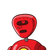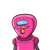# (11) -10 is greater than -7,(11) Zero is larger than every negative integer,(iv) The sum of two negative integers is a

(11) -10 is greater than -7,
(11) Zero is larger than every negative integer,
(iv) The sum of two negative integers is a negative integer
,
(v) The sum of a negative integer and a positive integer is always​

### 2 thoughts on “(11) -10 is greater than -7,<br />(11) Zero is larger than every negative integer,<br />(iv) The sum of two negative integers is a”

1.Step-by-step explanation:

false

true

false

false, it depends on the numbers if the negative integer is larger then the sum is a negative integer and if positive integer is larger then the sum will become positive. therefore it doesn’t become always

for example,-4+2=-2 and -2+4=2

2.